## Introduction¶

As is commonly known to most Data Science practitioners and researchers, a Decision Tree splits the input space into mutually exclusive subsets. To predict unseen data, the mean of the target variable in a subset gets assigned as a prediction for each future instance in the respective subspace.

To construct the subspaces, the standard Decision Tree applies a greedy search algorithm to find the optimal binary split at each step. A general advantage of Decision Trees is their fair robustness against pertubations of the inputs. Actually, they even benefit from random pertubations in Ensemble methods as we all know from Random Forests and its siblings.

Today’s idea also builds on a split of data into mutually exclusive subsets, but builds those subsets almost completely at random.

## Data and preprocessing¶

I reused the housing prices dataset from a past post as it allows to nicely plot the results with some geographic intuition.

In :
from sklearn.cluster import KMeans, k_means

import pandas as pd
import numpy as np
import itertools

from sklearn.model_selection import train_test_split

from sklearn.linear_model import Lasso, Ridge, ElasticNet, LinearRegression
from sklearn.ensemble import RandomForestRegressor
from sklearn.neighbors import KNeighborsRegressor
from sklearn.tree import DecisionTreeRegressor

import matplotlib.pyplot as plt

from sklearn.preprocessing import StandardScaler

from copy import deepcopy

from sklearn.preprocessing import PolynomialFeatures

In :
df = pd.read_csv("housing.csv")

In :
df.head()

Out:
longitude latitude housing_median_age total_rooms total_bedrooms population households median_income median_house_value ocean_proximity
0 -122.23 37.88 41.0 880.0 129.0 322.0 126.0 8.3252 452600.0 NEAR BAY
1 -122.22 37.86 21.0 7099.0 1106.0 2401.0 1138.0 8.3014 358500.0 NEAR BAY
2 -122.24 37.85 52.0 1467.0 190.0 496.0 177.0 7.2574 352100.0 NEAR BAY
3 -122.25 37.85 52.0 1274.0 235.0 558.0 219.0 5.6431 341300.0 NEAR BAY
4 -122.25 37.85 52.0 1627.0 280.0 565.0 259.0 3.8462 342200.0 NEAR BAY

To keep things simple and visualizable, we only try to predict median house value from geographic location. A lot of the other variables are likely rather correlated with location and therefore might not give much additional information anyway.

In :
np.random.seed(123)
df = df[["longitude","latitude","median_house_value"]]

X = df.drop("median_house_value",1)
y = pd.DataFrame(df["median_house_value"])

In :
xx = np.ravel(X.values[:,0])
yy = np.ravel(X.values[:,1])
zz = np.ravel(y.values)

plt.figure(figsize = (10,8))
plt.scatter(xx,yy,c=zz,cmap = plt.cm.coolwarm.reversed(), s = 4)
plt.colorbar()

Out:
<matplotlib.colorbar.Colorbar at 0x1132a9c18>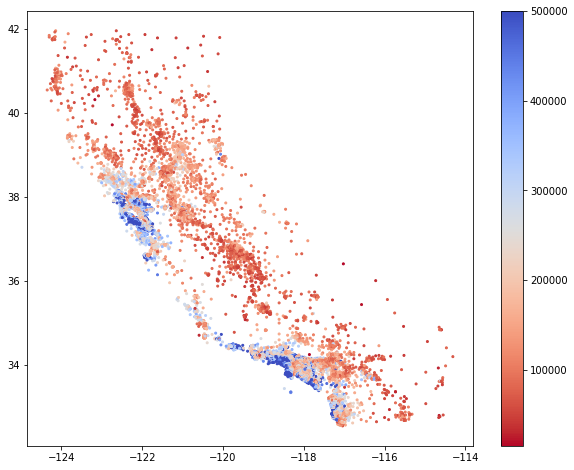In :
#train test split
X_train, X_test, y_train, y_test = train_test_split(X,y, test_size = 0.5,random_state = 23)

In :
scaler = StandardScaler()

X_train = scaler.fit_transform(X_train)
X_test = scaler.transform(X_test)


## The model¶

Instead of finding the optimal split at each tree node, the subsets get defined (almost) completely at random. The only parameter that needs to be set beforehand, is the number of desired subsets $S$. This point makes the proposed method a bit less adaptive than Decision Trees. As we will see, this does not necessarily make the approach less performant. The base algorithm itself is pretty simple:

1) Once we got $S$ set, $S$ different input feature vectors $X_s\, (s\in{1,…,S}$) are sampled at random from the training data.

2) Then, perform K-Means clustering, using the $X_s$’s as the cluster means.

3) Finally, calculate the means $\bar{y}_s\, (s\in{1,…,S}$ of the target variable in each cluster.

4) To estimate an unseen datapoint $\tilde{X}$, predict the corresponding cluster $\tilde{s}$ based on K-Means and use the respective mean as a prediction $\tilde{y}=\bar{y}_\tilde{s}$ as you would do in a Regression Tree

I wrote a possible implementation below:

In :
class ClusterRegressor:

def fit(self, X,y, n_cluster = 1000):

X_n = pd.DataFrame(X)
y_n = pd.Series(y)

rows = np.random.choice(np.arange(X_n.shape),n_cluster,replace = False)

self.km = KMeans(n_cluster,init = X_n.iloc[rows,:].values, n_init = 1, max_iter = 1, n_jobs = -1)
self.single_ys = y_n.iloc[rows]

self.labels = pd.Series(self.km.fit_predict(X_n), index = X_n.index)

self.means = pd.Series(np.nan, self.labels.unique())

for label in self.means.index.tolist():

indexes = self.labels.loc[self.labels==label].index

self.means.loc[label] = y_n.loc[indexes].mean()

def predict(self, X):

X_n = pd.DataFrame(X)
preds = pd.Series(np.nan, index = X_n.index)

labels = pd.Series(self.km.predict(X_n), index = X_n.index)

for label in self.means.index.tolist():

indexes = labels.loc[labels==label].index
preds.loc[indexes] = self.means.loc[label]

if preds.isnull().any():
for nanindex in preds[preds.isnull()].index:
nanlabel = labels.loc[nanindex]
preds.loc[nanindex] = self.single_ys.iloc[nanlabel]

return preds.values


Finding an optimal number of clusters is a bottleneck that needs to be solved e.g. via cross-validation. For this example I used an arbitrary amount of 1000 clusters.

In :
np.random.seed(123)

ct = ClusterRegressor()

ct.fit(X_train, y_train.values.reshape(-1),1000)

pred = ct.predict(X_test)

rmse_kmk = np.sqrt(np.mean((pred-y_test.values.reshape(-1))**2))


Let’s see how the algorithm performs in comparison to a Decision Tree:

In :
tr = DecisionTreeRegressor()
tr.fit(X_train,y_train)

pred_tr = tr.predict(X_test).reshape(-1)
rmse_tr = np.sqrt(np.mean((pred_tr-y_test.values.reshape(-1))**2))

In :
print(rmse_kmk)
print(rmse_tr)

63472.96053216668
65061.9441001864


Personally, I find it remarkable that a more or less completely random assignment of subsets can keep up with an optimized regression tree. Of course this is no proof that the method is superior – especially higher dimensions will probably be more difficult to deal with.

Nevertheless, let’s visualize:

In :
X_coordinates = np.linspace(df.values[:,0].min(),df.values[:,0].max(), 500).reshape(-1,1)
Y_coordinates = np.linspace(df.values[:,1].min(),df.values[:,1].max(), 500).reshape(-1,1)

xx_grid, yy_grid = np.meshgrid(X_coordinates, Y_coordinates)

point_space = np.c_[xx_grid.ravel(), yy_grid.ravel()]
point_space = scaler.transform(point_space)

In :
pred = ct.predict(point_space)

contour_predictions = pred

plt.figure(figsize = (10,8))

plt.imshow(contour_predictions.reshape(500,500), cmap=plt.cm.coolwarm.reversed(), origin='lower',
extent=[np.min(X_coordinates), np.max(X_coordinates), np.min(Y_coordinates), np.max(Y_coordinates)]
)
xx = np.ravel(X.values[:,0])
yy = np.ravel(X.values[:,1])
zz = np.ravel(y.values)

plt.colorbar()

Out:
<matplotlib.colorbar.Colorbar at 0x1138c31d0>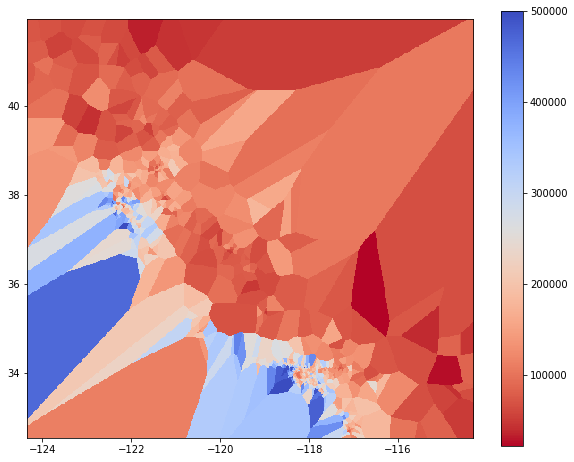Cool thing is that contary to Decision Trees, the resulting subsets are no hypercubes but are adaptive to the training data they are built on. This can however also lead to overfitting more easily.

## The next logical step: Build an ensemble¶

Due to its relation to Decision Trees, I was curious how the approach does in a Random Forest-like ensemble. Below is an implementation that follows the same steps as a simple Random Forest by replacing the Decision Trees with the randomized subset algorithm:

In :
class ClusterForest:

def __init__(self, n_estimators=25):

self.n_estimators = n_estimators

def fit(self, X, y, n_cluster = 1000):

self.estimators = []

for i in range(self.n_estimators):

#Baggin is also implemented
draws = np.random.choice(np.arange(len(X)), len(X), replace = True)

ct = ClusterRegressor()
ct.fit(np.array(X)[draws,:],np.array(y)[draws], n_cluster)
self.estimators.append(ct)

def predict(self, X):

result = []

for i in range(self.n_estimators):
result.append(self.estimators[i].predict(X).reshape(-1,1))

return np.mean(np.concatenate(result,1),1)

In :
np.random.seed(123)

cf = ClusterForest(25)
cf.fit(X_train, y_train.values.reshape(-1),2500)
pred_cf = cf.predict(X_test)

rmse_cf = np.sqrt(np.mean((pred_cf-y_test.values.reshape(-1))**2))


Let’s compare that to a Random Forest:

In :
rf = RandomForestRegressor(random_state = 123, n_estimators = 25)
rf.fit(X_train,y_train.values.reshape(-1))

pred_rf = rf.predict(X_test).reshape(-1)
rmse_rf = np.sqrt(np.mean((pred_rf-y_test.values.reshape(-1))**2))

In :
print(rmse_cf)
print(rmse_rf)

53203.59329595321
54612.78650551102


Again, the result outperforms the Tree version. The disadvantage however is that we loose the variable selection feature of Trees. An interesting extension would therefore be a feature bagging before applying K-Means.

Ensemble visualization:

In :
pred = cf.predict(point_space)

contour_predictions = pred

plt.figure(figsize = (10,8))

plt.imshow(contour_predictions.reshape(500,500), cmap=plt.cm.coolwarm.reversed(), origin='lower',
extent=[np.min(X_coordinates), np.max(X_coordinates), np.min(Y_coordinates), np.max(Y_coordinates)]
)
xx = np.ravel(X.values[:,0])
yy = np.ravel(X.values[:,1])
zz = np.ravel(y.values)

plt.colorbar()

Out:
<matplotlib.colorbar.Colorbar at 0x1137eb080>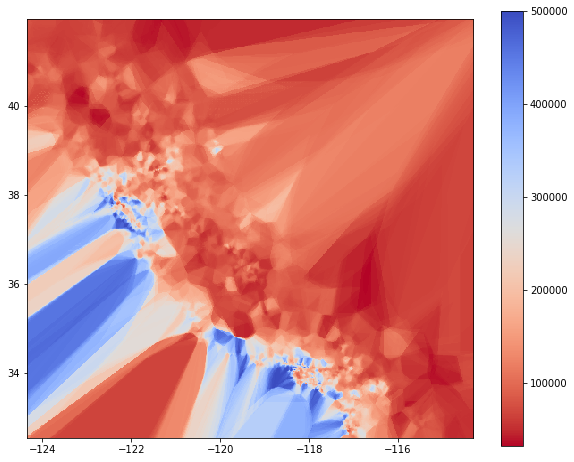## Going further with Piecewise Linear Regression¶

I am a big fan of interpretable Machine Learning and after some reasonning, the following idea came up to me:

Instead of simply using the mean, build a (penalized) Linear Regression model on each subset. For an unseen datapoint, we simply predict using the linear model corresponding to the respective cluster.

The implementation is then pretty straightforward again:

In :
class ClusterRegressorLin:

def fit(self, X,y, n_cluster = 2000):

X_n = pd.DataFrame(X)
y_n = pd.Series(y)

rows = np.random.choice(np.arange(X_n.shape),n_cluster,replace = False)

self.km = KMeans(n_cluster,init = X_n.iloc[rows,:].values, n_init = 1, max_iter = 1, n_jobs = -1)
self.single_ys = y_n.iloc[rows]

self.labels = pd.Series(self.km.fit_predict(X_n), index = X_n.index)

self.means = pd.Series(np.nan, self.labels.unique())
self.models = {}

for label in self.means.index.tolist():

indexes = self.labels.loc[self.labels==label].index

model = Ridge()
model.fit(X_n.loc[indexes],y_n.loc[indexes])
self.models[label] = model

self.means.loc[label] = y_n.loc[indexes].mean()

def predict(self, X):

X_n = pd.DataFrame(X)
preds = pd.Series(np.nan, index = X_n.index)

labels = pd.Series(self.km.predict(X_n), index = X_n.index)

for label in self.means.index.tolist():

indexes = labels.loc[labels==label].index

if len(X_n.loc[indexes,:])>0:

if self.models[label] is not None:
#try:
preds.loc[indexes] = self.models[label].predict(X_n.loc[indexes,:])
#except:
#    preds.loc[indexes] = self.means.loc[label]
else:
preds.loc[indexes] = self.means.loc[label]

if preds.isnull().any():
for nanindex in preds[preds.isnull()].index:
nanlabel = labels.loc[nanindex]
preds.loc[nanindex] = self.single_ys.iloc[nanlabel]

return preds.values


Let’s check the performance:

In :
np.random.seed(123)

ctl = ClusterRegressorLin()

ctl.fit(X_train, y_train.values.reshape(-1),2000)

pred_ctl = ctl.predict(X_test)

rmse_ctl = np.sqrt(np.mean((pred_ctl-y_test.values.reshape(-1))**2))

print(rmse_ctl)

60068.47074963777


…and visualize again:

In :
np.random.seed(123)

pred = ctl.predict(point_space)

contour_predictions = pred

plt.figure(figsize = (10,8))

plt.imshow(contour_predictions.reshape(500,500), cmap=plt.cm.coolwarm.reversed(), origin='lower',
extent=[np.min(X_coordinates), np.max(X_coordinates), np.min(Y_coordinates), np.max(Y_coordinates)]
)
xx = np.ravel(X.values[:,0])
yy = np.ravel(X.values[:,1])
zz = np.ravel(y.values)

plt.colorbar()

Out:
<matplotlib.colorbar.Colorbar at 0x1a1c106470>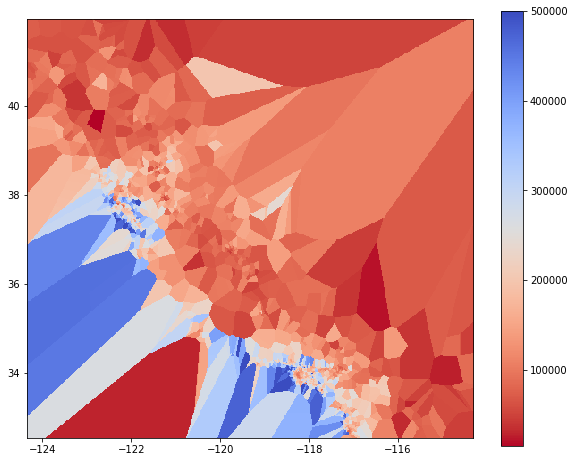Now we have a piecewise linear model which means we can interpret the prediction while having performance similar or even better to a fully grown Decision Tree.

## More ensembling¶

Of course, we can ensemble again in a Random Forest-like manner:

In :
class ClusterForestLin:

def __init__(self, n_estimators=25):

self.n_estimators = n_estimators

def fit(self, X, y, n_cluster = 2000):

self.estimators = []

for i in range(self.n_estimators):

draws = np.random.choice(np.arange(len(X)), len(X), replace = True)

ct = ClusterRegressorLin()
ct.fit(np.array(X)[draws,:],np.array(y)[draws], n_cluster)
self.estimators.append(ct)

def predict(self, X):

result = []

for i in range(self.n_estimators):
result.append(self.estimators[i].predict(X).reshape(-1,1))

return np.mean(np.concatenate(result,1),1)


I went down a little on the amount of clusters since the linear model introduces some more degrees of freedom which could lead to overfitting.

In :
np.random.seed(123)

cf = ClusterForestLin(25)
cf.fit(X_train, y_train.values.reshape(-1))
pred_cf = cf.predict(X_test)

rmse_cf = np.sqrt(np.mean((pred_cf-y_test.values.reshape(-1))**2))

print(rmse_cf)

53597.58298520474


Since each forecast is the average of $S$ linear models we can simply average the coefficients of all models for a given prediction. We then have a fully interpretable, piecewise linear model that performs comparably well as a non-interpretable Random Forest.

Of course, variable selection will likely become an issue so the performance on this example should be taken with some grain of salt.

This can probably be solved with some finetuning. Since the approach is parallelizable, we can also build complex ensembles rather easy.

Let’s do a final visualization:

In :
pred = cf.predict(point_space)

contour_predictions = pred

plt.figure(figsize = (10,8))

plt.imshow(contour_predictions.reshape(500,500), cmap=plt.cm.coolwarm.reversed(), origin='lower',
extent=[np.min(X_coordinates), np.max(X_coordinates), np.min(Y_coordinates), np.max(Y_coordinates)]
)
xx = np.ravel(X.values[:,0])
yy = np.ravel(X.values[:,1])
zz = np.ravel(y.values)

plt.colorbar()

Out:
<matplotlib.colorbar.Colorbar at 0x1a21c0a080>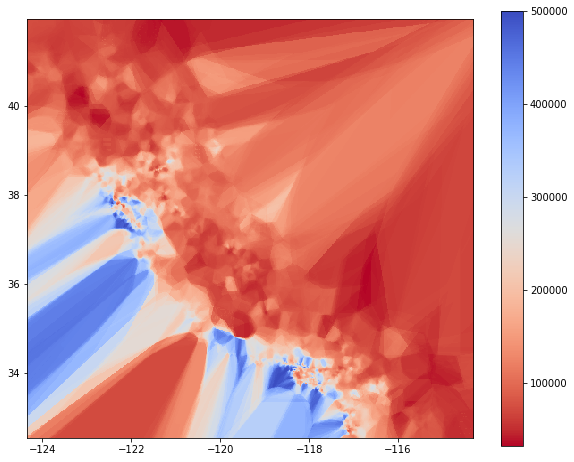## Conclusion¶

Decision Trees and their Ensemble extensions are powerful tools to use in predictive modeling. It is remarkable that we can achieve similar performance with a more or less completely random alternative. Although the approch is not perfect, the results are interesting nonetheless.

Especially the possibility to work with piecewise linear models in this framework and the resulting interpretability makes the whole thing promising to me. Both K-Means and the proposed ensembling are scalable so this could potentially be an interpretable and non-linear model that is also usable for big-data problems.